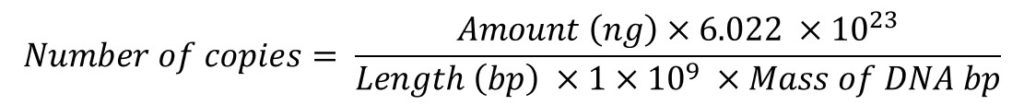# DNA Copy Number Online Calculator

Below is a calculator that can be used to estimate the number of copies of DNA, by simply entering the amount used and the length of DNA. DNA copy number calculations are an important step for absolute qPCR techniques during the standard curve creation.

It may also be worth checking out the guide on how to calculate the number of copies of DNA for qPCR for a more detailed look at how the equation works.

Once entered, hit the calculate button and the number of copies of DNA will be returned.

## How to use the calculator

1. Enter the amount of DNA, in nanograms (ng), and enter this into the first cell.

2. Enter the length of DNA, in base pairs (bp), and enter this in the second cell.

3. Select either single-stranded DNA (ssDNA) or double-stranded DNA (dsDNA) as the DNA type.

4. Click the calculate button.

5. The calculator will determine and return the number of copies of DNA.

Note: If you are using this calculator to determine the number of copies for DNA in qPCR, then select the dsDNA option, since the DNA will be double-stranded after PCR.

## How the calculator works

The DNA copy number calculator works by using the following equation.Further information for each component of the DNA copy number calculator is also listed below.

• Amount (ng) – The amount of DNA, in nanograms (ng), in the tube.
• 6.022 x 1023 – Avogadro’s constant. This represents the number of molecules per mole.
• Length (bp) – The length of DNA, in base pairs (bp), in the template.
• 1 x 109 – A factor used to convert to ng.
• Mass of DNA bp – The average mass of a DNA bp is either 660 (dsDNA) or 330 (ssDNA) g/mole. This value depends on what is selected as the type of DNA in the calculator.